45 Degree Angle - Definition with Examples

The Complete K-5 Math Learning Program Built for Your Child

• 30 Million Kids

Loved by kids and parent worldwide

• 50,000 Schools

Trusted by teachers across schools

• Comprehensive Curriculum

Aligned to Common Core

45-Degree Angle

When two rays intersect at a common endpoint, they form an angle. The common endpoint is called the vertex, and the rays are called the arms of the angle.An angle is measured in° or radians. If the two arms of an angle extend in exactly opposite directions, it is a straight angle. A straight angle measures 180°. An angle can be measured using a protractor, and the angle of measure 90 degrees is called a right angle. In a right angle, the two arms are perpendicular to each other.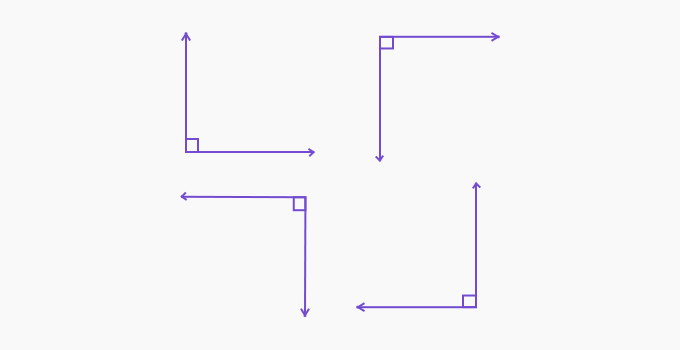When the right angle is divided into two equal parts each angle measures 45°.Constructing a angle using a protractor

Step 1: Draw a ray and name it AB.Step 2: Keep the center point of the protractor at A. Since the angle opens to the right, choose 45° in the list that starts at the right and moves in the anticlockwise direction. Mark the point C.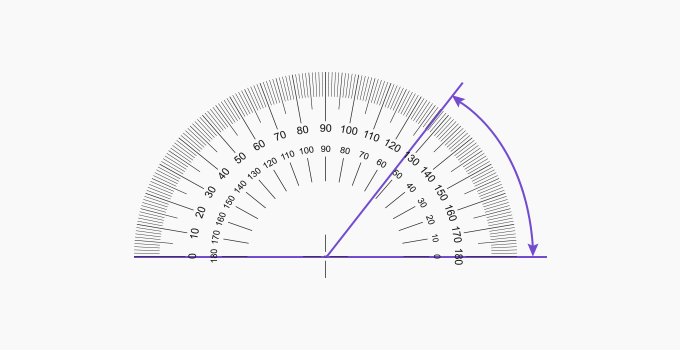Step 3: Join A and C. Here, measures .45 Degree Angles in Real Life:

• Symmetric windows

• Based on the location and climate of the place, some solar panels are installed at an angle of 45° for optimal solar energy productionConstructing a angle using a compass

Step 1: Draw a line segment  and the perpendicular bisector of  by intersecting the arcs of radius more than half of the length MN. Let the perpendicular bisector intersect the line segment  at O.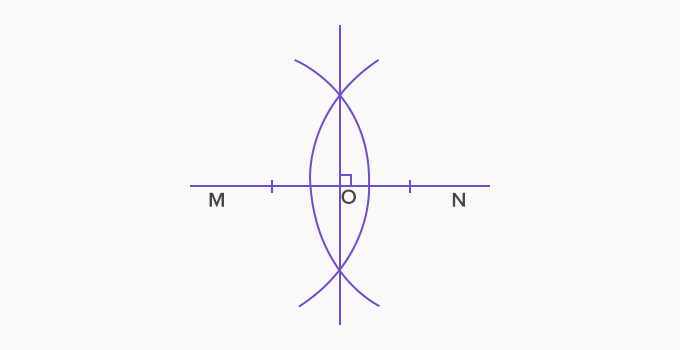Step 2: Draw an arc centered at O with a radius OM, cutting the perpendicular bisector at P.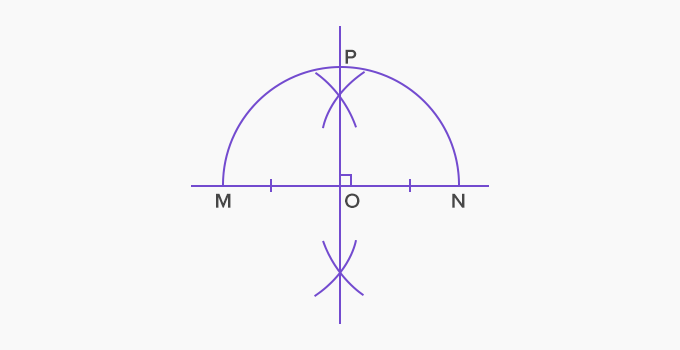Step 3: Join M and P using a straight line. measures .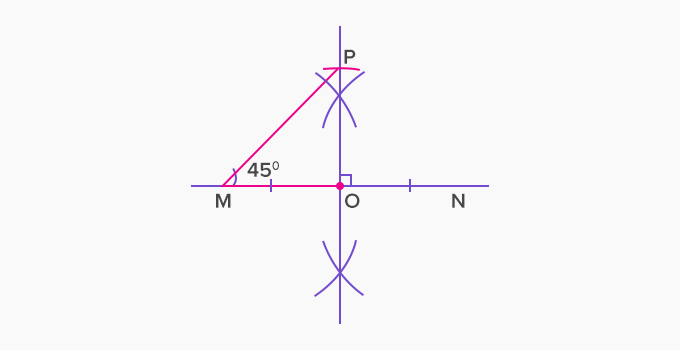An angle of measure  degree can also be constructed by constructing the angular bisector of a right angle.

 Fun Facts The diagonals of a square make an angle of measure  with the sides. If one acute angle of an isosceles right triangle measures , so is the other.

Won Numerous Awards & Honors